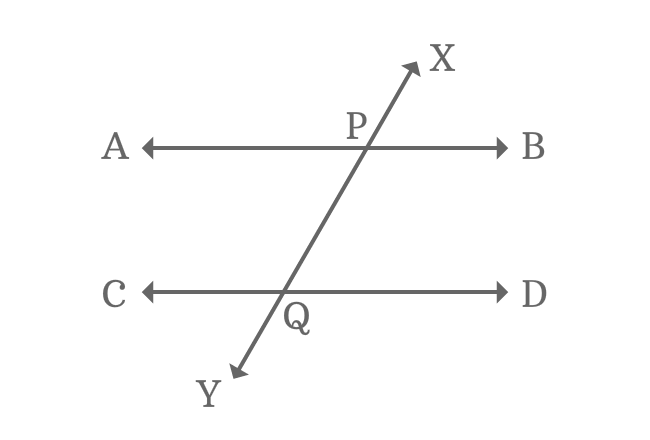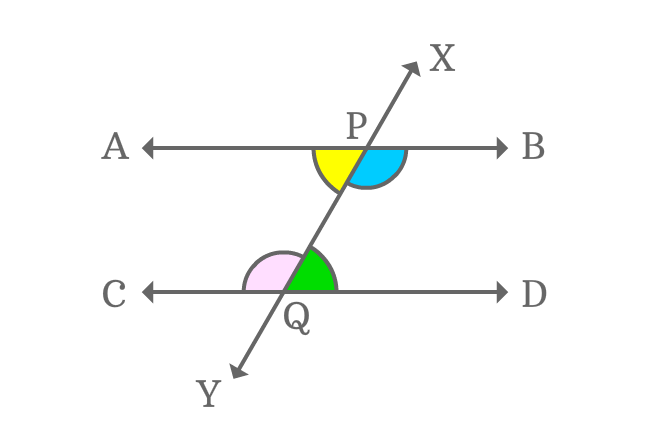# Alternate Interior Angles formed by Parallel Lines and their transversalThe interior angles which appear opposite sides of transversal of parallel lines are called alternate interior angles formed by parallel lines and their transversal.

When two parallel lines are cut by a transversal, four interior angles are formed geometrically and every two interior angles are appeared oppositely at the intersection of the parallel lines by their transversal.

Thus, two pairs of alternate interior angles are formed when two parallel lines are intersected by their transversal.

## Alternate Interior Angles$\angle APY$, $\angle BPY$, $\angle CQX$ and $\angle DQX$ are four interior angles, which are actually formed by the intersection of two parallel lines ($\overleftrightarrow{AB}$ and $\overleftrightarrow{CD}$) by their transversal line $\overleftrightarrow{XY}$.

$1. \,\,\,\,\,\,$ $\angle APY$ and $\angle DQX$

$2. \,\,\,\,\,\,$ $\angle BPY$ and $\angle CQX$

The above two pairs of angles are interior angles and also appear opposite sides of the transversal. Therefore, they are called alternate interior angles.

### PropertyThe every pair of alternate interior angles are equal geometrically when two parallel lines are intersected by their transversal line. It is possible because of the parallelism of the parallel lines.

$1. \,\,\,\,\,\,$ $\angle APY \,=\, \angle DQX$

$2. \,\,\,\,\,\,$ $\angle BPY \,=\, \angle CQX$

Latest Math Topics
Jun 26, 2023
Jun 23, 2023

###### Math Questions

The math problems with solutions to learn how to solve a problem.

Learn solutions

Practice now

###### Math Videos

The math videos tutorials with visual graphics to learn every concept.

Watch now

###### Subscribe us

Get the latest math updates from the Math Doubts by subscribing us.